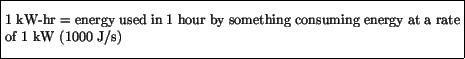Next: Other types of energy Up: Potential Energy Previous: Elastic or Spring Potential

## Electrical Potential Energy

A third type of potential energy we mention is that due to the electrical force - this is the force of attraction between electric charges that causes that annoying static cling in your dryer. We will discuss this in more detail in Chapter 9 - for now, we only mention that the voltage'' of a car battery or household outlet is actually a measure of the electrical potential energy between two terminals.

On your electric bill one can find the number of kilowatt-hours'' of electricity that has been used in a month - this is a measure of the electrical energy that has been consumed. A kilowatt-hour (kW-hr) is defined asAccording to this definition, we see that 1 kW-hr is a unit of energy, which can be expressed in Joules by multiplying the power (1000 W) by the time (1 hour = 3600 seconds) so thatThus, for example, burning a 120 W light bulb for 100 hours will consume 120 W x ( 1 kW / 1000 W ) x 100 hr = 12 kW-hr, which is about 43 million Joules. If electricity is charged at a rate of $0.08 per kW-hr, this would cost 12 x$0.08 = \$0.96. Clearly the cost of electricity would seem even more reasonable if expressed in terms of Joules.Next: Other types of energy Up: Potential Energy Previous: Elastic or Spring Potential
modtech@theory.uwinnipeg.ca
1999-09-29